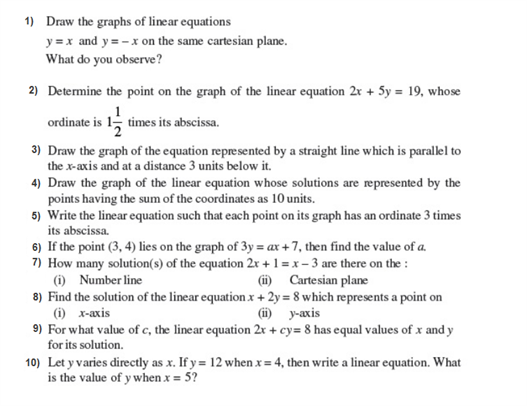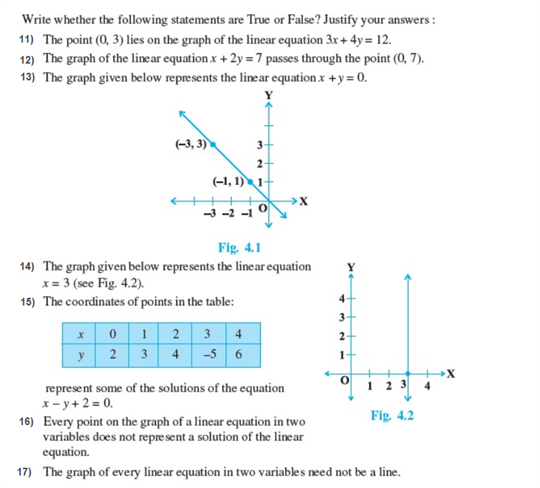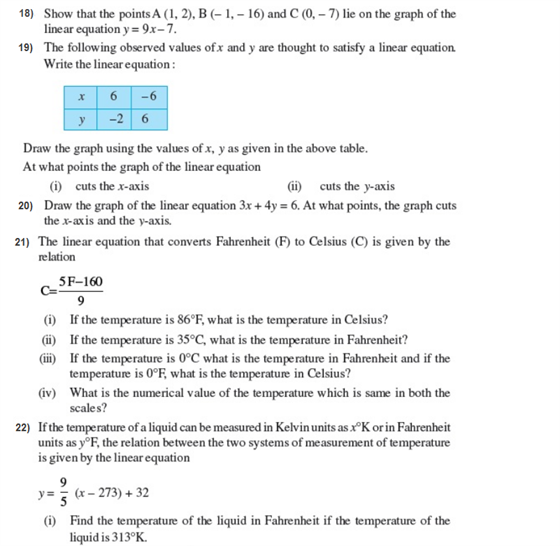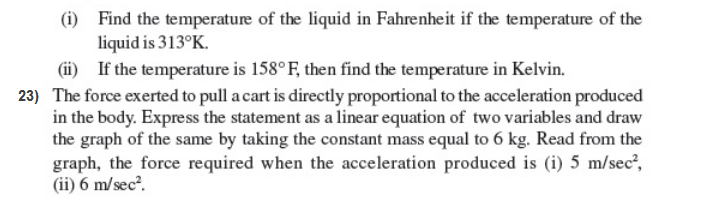Chapter 4: Linear Equations in Two VariablesPractise This Question

If y = 4x is rewritten in the form ax + by + c = 0, which of these options represents the values of a,b and c respectively?